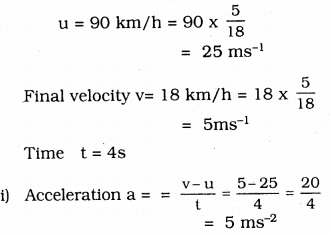# KSEEB Solutions for Class 9 Science Chapter 9 Force and Laws of Motion

## Karnataka Board Class 9 Science Chapter 9 Force and Laws of Motion

### KSEEB Solutions Class 9 Science Chapter 9 Intext Questions

Question 1.
Which of the following has more inertia
(a) a rubber ball and a stone of the same size?
(b) a bicycle and a train?
(c) a five rupees coin, and a one rupee coin?
a) a stone
b) a train,
c) Five rupees coin.

Question 2.
In the following example, try to identify the number of times the velocity of the ball changes:
“A football player kicks a football to another player of his team who kicks the football towards the goal. The goalkeeper of the opposite team collects the football and kicks it towards a player of his own team.”
Also, identify the agent supplying the force in each case.
Given below are the number of times at which the velocity of the ball changes. Whenever a force will be applied on the ball then the velocity of the ball will change.

1. When the first player kicks the ball towards another player of his team the velocity of the ball will change because the first player applied some force on the ball.
2. When another player kicks the ball towards the goal, then the velocity of the ball will change, here again, the force is applied on the ball.
3. When goalkeeper of the opposite team collects the ball, then the velocity of ball change. It becomes zero. Here the goalkeeper applies some force on the ball to stop.
4. When the goalkeeper kicks the ball towards his own team, the velocity of the ball changes because the goalkeeper applies some force on the ball.

Question 3.
Explain why some of the leaves may get detached from a tree we vigorously shake its branch.
We know that the leaves are attached to the tree and are in a state of rest initially. But when the tree is vigorously shaken, the branches of the tree come in the state of motion but the leaves tend to maintain their state of rest, as a result, the leaves get detached from the tree and fall down.

Question 4.
Why do you fall in the forward direction when a moving bus brakes to a stop and fall back when it accelerates from rest?
Initially, when the bus is at rest our body also follows the same state. But all of a sudden when the bus starts to move the lower part of our body tends to move with the motion of the bus but the upper part rejects this state of motion and continues to be in a state of rest. This results in a sudden jerk backward when a bus moves. Also, a person standing in a bus will be in a state of motion, and when the brakes are applied the lower part of our body comes to the state of rest but our upper part is in state of motion. Hence, we tend to fall forward when the bus applies brakes.

Question 5.
If action is always equal to the reaction, explain how a horse can pull a cart.
In order to pull the cart, the horse pushes the ground with its foot in the backward direction by pressing the ground. As a reaction of this force, the ground pushes the horse in a forward direction. As a result, the cart is pulled by the horse in the forward direction.

Question 6.
Explain Why is it difficult for a firement to hold a hose, which ejects large amounts of water at a high velocity.
From Newton’s third law, we know that every action has an equal and opposite reaction. So when a fireman holds a hose-pipe which is ejecting a large amount of water at high-velocity experiences a backward push due to the force of water flowing in the forward direction. Hence making it clear that due to the action of force in the forward direction the force is applied on the pipe in the backward direction, thus making the fireman difficult to hold the hose-pipe.

Question 7.
From a rifle of mass 4 kg, a bullet of mass 50 g is fired with an initial velocity of 35 ms-1, calculate the initial recoil velocity of the rifle.
Mass of the gun M = 4 kg.
Mass of bullet M = 50 g = 50 × 10-3kg.
Initial velocity of the bullet v = 35ms-1
Let recoil velocity of the gun be vms-1
Before firing the bullet both gun and bullet were in rest so total momentum of gun and bullet is zero, After firing
Momentum of bullet = mv,
Momentum of gun = Mv
Total momentum of bullet and gun after firing = mv + Mv
Since there is no external force applied to the system.
So total momentum after firing = total momentum before firing
mv + Mv = 0
Mv = -mvHere negative sign shows that there recoil velocity of the gun is in the direction opposite to the velocity of the bullet.

Question 8.
Two objects of masses 100 g and 200 g are moving along the same line and direction with velocities of 2ms-1 and 1ms-1 respectively. They collide and after the collision, the first object moves at a velocity of 1.67ms-1. Determine the velocity of the second object.
Before collisionAfter collision
m1 = 100 g = 0.1 Kg
m2 = 200 g = 0.2 Kg
V1 = 1.67 ms-1
Let velocity of second object after collision is v2ms-1 Since there is no external force on the system.

### KSEEB Solutions for Class 9 Science Chapter 9 Textbook Exercises

Question 1.
An object experiences a zero external unbalanced force. Is it possible for the object to be travelling with a non-zero velocity? If yes, state the conditions that must be placed on the magnitude and direction of the velocity. If no provide a reason.
According to Newton’s 1st law of motion, no net force is required to move an object which is moving with a constant velocity. So when an object experiences a net zero external unbalanced force, then it can move with a non-zero velocity. Also if an object is initially at rest not net force acts upon it, thus the object may not move all.

Question 2.
When a carpet is beaten with a stick, dust comes out of it. Explain.
When a carpet is beaten with a stick, then the fibers of the carpet attain the state of motion while the dust particles remain in rest due to the inertia of rest. Therefore the dust particles fall down.

Question 3.
Why is it advised to tie any luggage kept on the roof of a bus with a rope?
Because even though bus comes to rest, luggage are in motion, They fall down. So, it is tied with a rope to prevent falling.

Question 4.
A batsman hits a cricket ball which then rolls on level ground. After covering a short distance, the ball comes to rest. The ball slows to a stop because.
a) The batsman did not hit the ball hard enough.
b) Velocity is proportional to the force exerted on the ball.
c) There is a force on the ball opposing the motion.
d) There is no unbalanced force on the ball, so the ball would want to come to rest.
c) because the ball slows down to rest as the force of friction acting between the ground and the ball opposes the motion of the ball.

Question 5.
A truck starts from rest and rolls down a hill with a constant acceleration. It travels a distance of 400m in 20 s. Find its acceleration. Find the force acting on it if its mass is 7 tons
(Hint: 1 ton = 1000 kg.)
The truck starts from rest, so initial velocity u = 0
Distance s = 400 m, t = 20s
m = 7 tons = 7 × 1000 = 7000 Kg.
From the 2nd equation of motion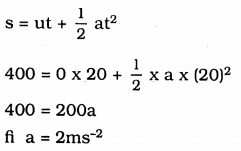From Newton’s 2nd law of motion, the force acting on the Duck
= 7000 × 2
= 14000 N
= 1.4 × 104 N.

Question 6.
A stone of 1 kg is thrown with a velocity of 20ms-1 across the frozen surface of a lake and comes to rest after travelling a distance of 50 m. What is the force of friction between the stone and the ice?
Mass of the stone m = 1 kg.
initial velocity u = 20 ms-1
Final velocity v = 0 (Therefore the stone comes to rest distance travelled S = 50 m)
From third equation of motion
v2 = u2 + 2as
(0)2 = (20)2 + 2a(50)
100 a = -400
∴ a = -4ms-2
Here negative sign shows that there is relation in the motion of stone.
Force of friction between stone and ice = Force required to stop the stone
= ma
= 1 × -4 = -4N or 4N.

Question 7.
An 8000 kg engine pulls a train of 5 wagons, each of 2000 kg along a horizontal track. If the engine exerts a force of 40000 N and the track offers a friction force of 5000N then calculate
a) the net accelerating force.
b) the acceleration of the train and
c) the force of wagon 1 on wagon 2
a) Net accelerating force = Force exerted by the engine – Frictional force.
(Here the frictional force is substracted because it opposes the motion)
= 40000 – 5000 = 35000 N
= 3.5 × 104 N.

b) From Newton’s second law of motion
Accelerating force = mass of the train × Acceleration of train
a =$$\frac{\mathrm{F}}{\mathrm{m}}$$
Mass of train = Mass of engine + Mass of all wagons
= 8000 + 5 × 2000
= 8000 + 10000
= 18000 Kg.
∴ Acceleration = $$\frac{35000}{18000}=\frac{35}{18}$$
= 1.95 ms-2.

c) Force of wagon 1 on wagon 2
= Mass of the engine × Acceleration
= 800 × 1.95 = 15600 N.

Question 8.
An automobile vehicle has a mass of 1500 Kg. what must be the force between the vehicle and road if the vehicle is to be stopped with a negative acceleration of 1.7 ms-2
Mass m = 1500 Kg.
Acceleration a = -1.7 ms-2
From Newton’s second law of motion
F = ma
= 1500 × (-1.7)
= -2550 N.

Question 9.
What is the momentum of an object of mass m, moving with a velocity V?
(a) (mv)2
(b) mv2
(c) $$\frac{1}{2}$$ mv2
(d) mv.
(d) P = mv.

Question 10.
Using a horizontal force of 200N, we intend to move a wooden cabinet across a floor at a constant velocity. What is the friction force that will be exerted on the cabinet?
The cabinet will move across the floor with constant velocity if there is no net external force of 200N that should be applied on the cabinet in opposite direction.
Thus, the frictional force = 200N
(Frictional force always acts in the direction opposite to the direction of motion)

Question 11.
Two objects, each of mass 1.5 kg are moving in the same straight line but in opposite directions. The velocity of each object is 2.5ms-1 before the collision during which they stick together is what will be the velocity of combined object after collision?
Mass of the first object, m1 = 1.5 Kg.
Mass of second object m2 = 1.5 Kg.
Velocity of first object before the collision, u1 = 2.5 ms-1
velocity of second object before collision, u2 = -2.5 ms-1
Here negative sign is taken because the second object is moving in the direction opposite to the direction of motion of first object.
Mass of combined object after collision
M = m1 + m2 (Since they stick together)
= 1.5 + 1.5 = 3 Kg.
Let velocity of combined object after collision be vms-2
Here there is no external force, so from law of conservation of momentum
Momentum after collision = Momentum before collision
mv = m1u1 + m2u2
3 × v = 1.5 × 2.5 + 1.5 (-2.5) =0
∴ v = 0.

Question 12.
According to the third law of motion when we push on an object, the object pushes back on us with an equal and opposite force. If the object is a massive truck parked along the roadside, it will probably not move. A student justifies this by answering that the two opposite and equal forces cancel each other. Comment on this logic and explain why the truck does not move.
The logic given by students is not correct because two equal and opposite forces cancel each other only in the case if they act on the somebody. According to Newton’s third law action and reaction force always act on two different bodies, so they cannot cancel each other. When a massive truck is pushed then the truck may not the force applied is not sufficient to move the truck.

Question 13.
A hockey ball of mass 200 g travelling at 10ms-1 is struck by a hockey stick so as to return it along its original path with a velocity at 5ms-1 Calculate the change of momentum occurred in the motion of the hockey ball by the force applied by the hockey stick.
Mass of hockey ball m = 200 g = 0.2 Kg
Initial velocity u = 10ms-1
Final velocity v = 5 ms-1
(Since the momentum of the ball = Final momentum – initial momentum
= mv – mu
= m (v – u)
= -0.2 (-5 – 10)
= 0.2 × -15 = -3 Kgms-1.

Question 14.
A bullet of mass 10 g travelling horizontally with a velocity of 150ms’1 strikes a stationary wooden block and comes to rest in 0.03s. Calculate the distance of penetration of the bullet into the block. Also, calculate the magnitude of the force exerted by the wooden block on the bullet.
Mass of bullet m = $$\frac{10}{1000}$$ = kg
= 0.001 Kg.
Initial velocity, u = 150ms-1
Final velocity v = 0 (since the bullet comes to rest)
Time t = 0.03 s.
From equation of motion
v = u + at
0 = 150 + a × 0.03
∴ a = $$\frac{-150}{0.03}$$
= -5000 ms-2
Magnitude of the force applied by the bullet on the block F = ma
= 0.01 × -5000 = -50N.

Question 15.
An object of mass 1Kg travelling in straight line with a velocity of 10ms’1 collides with, and sticks to, a stationary wooden block of mass 5 kg. Then they both move off together in the same straight line. Calculate the total momentum just before the impact and just after the impact Also calculates the velocity of the combined object.
Mass of object m1 = 1 Kg,
Velocity, u1 = 10ms-1
Mass of wooden block m2 = 5Kg,
velocity u2 = 0 (since wooden block is rest)
= 1 × 10 + 5 × 0 = 10 Kgms-1
According to law of conservation of momentum
Momentum after impact = Momentum before impact
(m1 + m2)v = 10
Where v = velocity of combined object
(1 + 5) v = 10
v = $$\frac{10}{6}$$ = 1.67ms-1.

Question 16.
An object of mass 100 Kg is accelerated uniformly from a velocity of 5ms-1 to 8ms-1 in 6s. Calculate the initial and final momentum of the object. Also find the magnitude of the force exerted on the object.
Mass of the object, m = 100 Kg
Initial velocity, u = 5 ms-1
Final velocity, v = 8 ms-2
(i) Initial momentum = mv = 100 × 5 = 500 Kgms-1
Final momentum = mv = 100 × 8 = 800 Kgms-1

(ii) From Newton’s second law
Force exerted on the object = Rate of change of momentum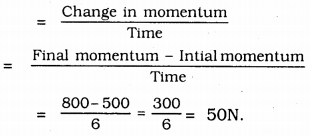Question 17.
Akhtar, Kiran, and Rahul were riding in a motorcar that was moving with a high velocity on an expressway when an insect hit the windshield and got stuck on the windscreen. Akhtar and Kiran started pondering over the situation. Kiran suggested that the insect suffered a greater change in momentum as compared to the momentum of the motor car (because the change in the velocity of the insect was much more than that of the motor car). Akthar said that since the motor car was moving with larger velocity, it exerted a larger force on the insect. And as a result, the insect died. Rahul while putting an entirely new explanation said that both the motor car and the insect experienced the same force and a change in their momentum. Comment on these suggestions.
The explanation given by Rahul is correct, as there is no external force on the system. So both the insect and motorcar experienced the same force and a change in momentum. .

Question 18.
How much momentum will a dumb-bell of mass 10 Kg transfer to the floor if it falls from a height of 80 cm? Take its downward acceleration to be 10ms-2.
m = 10 Kg, u = 0
Acceleration s = 80 cm = 0.8 m.
Velocity a = 10 ms-2
v2 = u2 + 2as
v2 = 0 + 2 × 10 × 0.8 =16
∴ v = $$\sqrt{16}$$ = 4ms-1
Momentum of dumb-bell just before it touches the floor
P = mv
= 10 × 4
= 40 Kgms-1.
When the dumbbell touches the floor its velocity becomes zero. Thus the total momentum of the dumbbell is transferred to the floor. Hence the momentum transferred to floor = 40 Kgms-2.

### KSEEB Solutions for Class 9 Science Chapter 9 Additional Questions and Answer

Question 1.
The following is the distance-time table of an object in motion.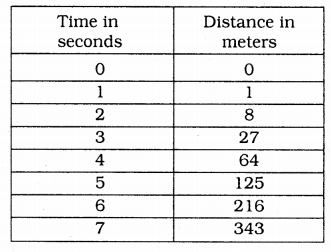a) What conclusion can you draw about the acceleration? Is it constant increasing, decreasing or zero?
b) What do you infer about the forces acting on the object?
a) Here, a = 0,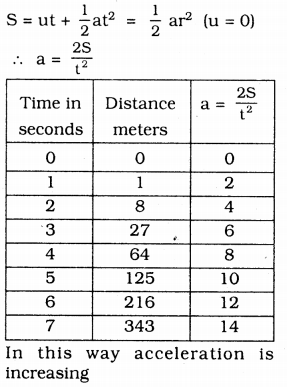In this way, acceleration is increasing

b) Since acceleration is increasing. So-net unbalanced force is acting on the object.

Question 2.
Two persons manage to push a motorcar of mass 1200 kg at a uniform velocity along a level road. The same motor car can be pushed by three persons to produce an acceleration of 0.2ms-2 with what force does each person push the motorcar? (Assume that all persons push the motorcar with the same muscular effort)
Mass of motor car m = 1200 Kg.
Acceleration produced a = 0.2 ms-2
Therefore force applied to the car by three persons = 1200 × 0.2 = 240 N.
∴ Force applied on the car by one person = $$\frac{240}{3}$$ = 80 N,

Question 3.
A hammer of mass 500g moving at 50ms-1 strikes a nail. The nail stops the hammer in a very short time of 0.61s what is the force of the nail on the hammer?
Mass of the hammer = 500g = 0.05 Kg.
Initial velocity of hammer u = 50 ms-1
The final velocity of the hammer, v = 0 (Since the hammer stops)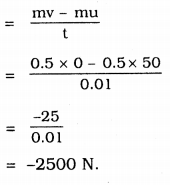Question 4.
A motor car of mass 1200 kg is moving along a straight line with a uniform velocity of 90km/h. Its velocity is slowed down to 18 km/h in 4s by an imbalanced external force. Calculate the acceleration and change in momentum. Also calculate the magnitude of the force required.
Mass m = 1200 kg.